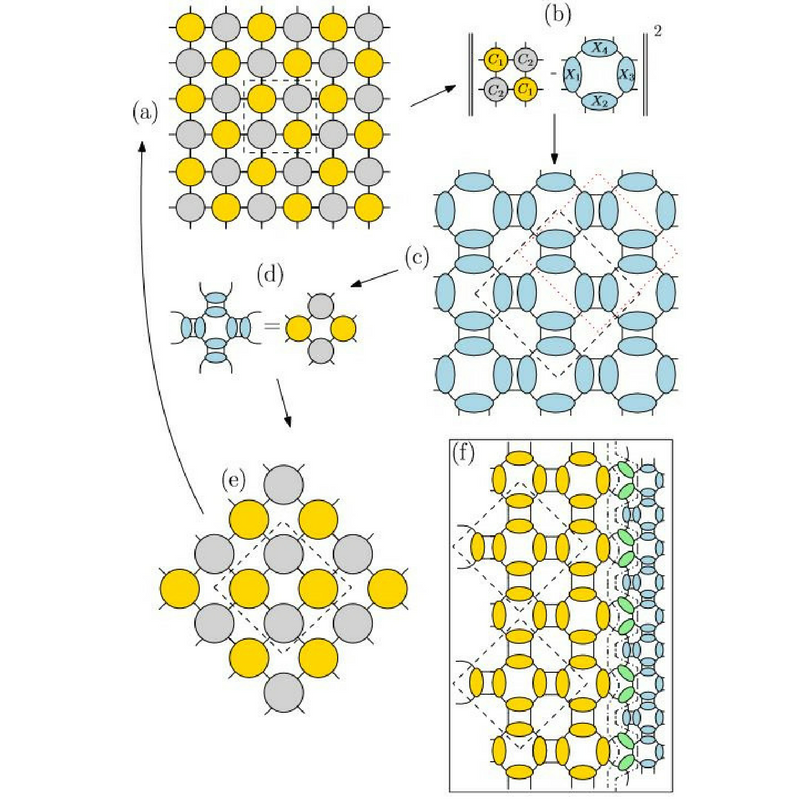# New Publication in Physical Review Letters

20.06.2017

Our publication on Renormalization Group Flows of Hamiltonians Using Tensor Networks was published in Physical Review Letters.

A renormalization group flow of Hamiltonians for two-dimensional classical partition functions is constructed using tensor networks. Similar to tensor network renormalization, we obtain approximate fixed point tensor networks at criticality. Our formalism, however, preserves positivity of the tensors at every step and hence yields an interpretation in terms of Hamiltonian flows. We emphasize that the key difference between tensor network approaches and Kadanoff's spin blocking method can be understood in terms of a change of the local basis at every decimation step, a property which is crucial to overcome the area law of mutual information. We derive algebraic relations for fixed point tensors, calculate critical exponents, and benchmark our method on the Ising model and the six-vertex model.

view full text: hereFIG.: (a)–(e) Illustration of a single step of the TNR+ algorithm. (a) Starting from a bipartite square lattice, (b) we approximate the periodic MPS representing a block of four sites by a rotated version (c) with a different tensor product structure and (d)contract these numerically optimized tensors exactly to (e) arrive at a coarse-grained tiltedlattice. (f) Iterating the TNR+ procedure in the presence of an open boundary generates a stochastic MERA.